# dotwhisker: Dot-and-Whisker Plots of Regression Results

#### 2017-06-28

Graphs have long been known to be a more compact and effective means of conveying the results of regression models than tables (Gelman, Pasarica, and Dodhia 2002; Kastellec and Leoni 2007), but many researchers continue to list these results in tables. The reason, Kastellec and Leoni (2007) surmised, is “simply put, it takes much greater effort to produce a quality graph than a table.” The dotwhisker package provides a quick and easy way to create highly customizable dot-and-whisker plots for presenting and comparing the output of regression models. It can be used to plot estimates of coefficients or other quantities of interest (e.g., predicted probabilities) within a single model or across different models: the estimates are presented as dots and their confidence intervals as whiskers (see Kastellec and Leoni 2007, 765–67).

Users can easily customize the content of their plots: presenting multiple models or results for a subset of variables is easy. Moreover, by outputting ggplot objects (Wickham 2009), dotwhisker allows users to further modify the format of their plots in nearly infinite ways.

This vignette illustrates basic use of the package’s mainstay function, dwplot, for creating dot-and-whisker plots from model objects; more advanced uses of dwplot that employ tidy data frames as input; and, finally, some useful variations of dot-and-whisker plots that are easily made using other functions in the dotwhisker package.

## Basic Use: Plotting Results from One or More Regression Models

Generating dot-and-whisker plots from model objects generated by the most commonly used regression functions is straightforward. To make a basic dot-and-whisker plot of any single model object of a class supported by broom::tidy, simply pass it to dwplot. For these examples, we’ll use the mtcars dataset extracted from the 1974 volume of the US magazine, Motor Trend.

#Package preload
library(dotwhisker)
library(broom)
library(dplyr)

# run a regression compatible with tidy
m1 <- lm(mpg ~ wt + cyl + disp + gear, data = mtcars)

# draw a dot-and-whisker plot
dwplot(m1)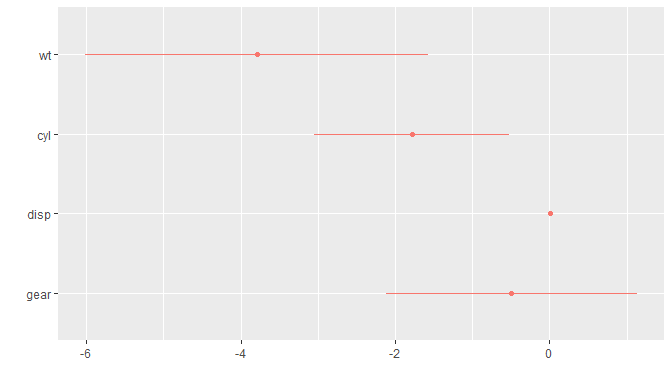By default, the whiskers span the 95% confidence interval. To change the width of the confidence interval, use the alpha argument:

dwplot(m1, alpha = .01)  # using 99% CIPlotting the results of more than one regression model is just as easy. Just pass the model objects to dwplot as a list. The dodge_size argument is used to adjust the space between the estimates of one variable when multiple models are presented in a single plot. Its default value of .15 will usually be fine, but, depending on the dimensions of the desired plot, more pleasing results may be achieved by setting dodge_size to lower values when the plotted results include a relatively small number of predictors or to higher values when many models appear on the same plot.

m2 <- update(m1, . ~ . + hp) # add another predictor
m3 <- update(m2, . ~ . + am) # and another

dwplot(list(m1, m2, m3))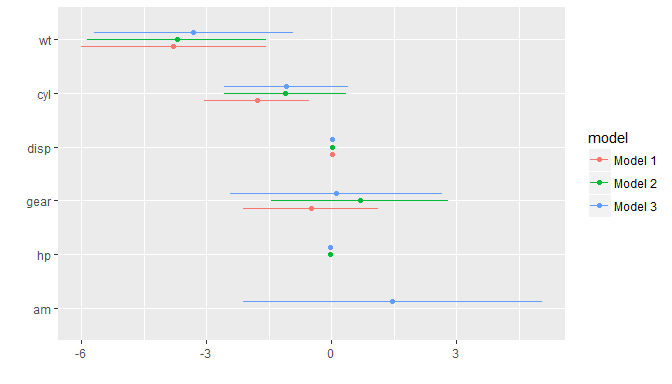dwplot also allows users to compare the result in a parallel way, as shown following.

dwplot(list(m1, m2, m3)) +
facet_grid(~model, scales="free_y")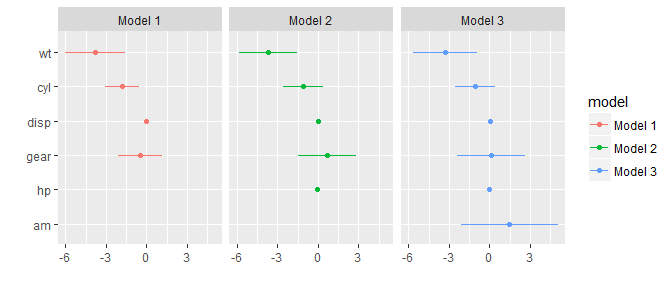Model intercepts are rarely theoretically interesting (see Kastellec and Leoni 2007, 765), so they are excluded by dwplot by default. They are easy to include if desired, however, by setting the show_intercept argument to true.

dwplot(list(m1, m2, m3), show_intercept = TRUE)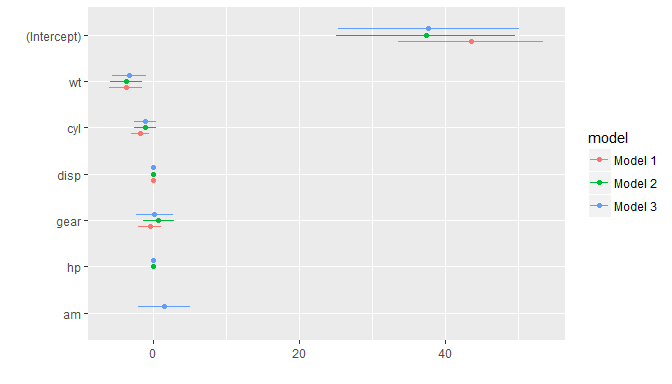Moreover, the output of dwplot is a ggplot object. Add or change any ggplot layers after calling dwplot to achieve the desired presentation.

dwplot(list(m1, m2, m3)) %>%
relabel_predictors(c(wt = "Weight",
cyl = "Cylinders",
disp = "Displacement",
hp = "Horsepower",
gear = "Gears",
am = "Manual")) +
theme_bw() + xlab("Coefficient Estimate") + ylab("") +
geom_vline(xintercept = 0, colour = "grey60", linetype = 2) +
ggtitle("Predicting Gas Mileage") +
theme(plot.title = element_text(face="bold"),
legend.justification=c(0, 0), legend.position=c(0, 0),
legend.background = element_rect(colour="grey80"),
legend.title = element_blank())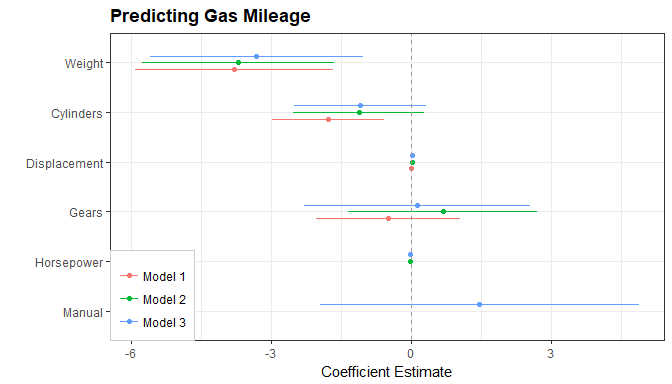Note that providing a named character vector to relabel_predictors, a dotwhisker function, conveniently renames the predictors.

There are many other packages (e.g., coefplot) that have the ability to draw dot-and-whisker plots of at least a single set of regression results taking model objects as input. While this is very convenient, it also comes with some severe limitations. First, many less common model objects are not supported. Second, rescaling coefficients, reordering them, or just plotting a subset of results is typically impossible. And third, quantities of interest beyond coefficient estimates cannot be plotted. The dotwhisker package avoids all of these limitations by optionally taking as its input a tidy data frame of estimates drawn from a model object rather than the model object itself.

## Advanced Use: Plotting Results Stored in a Tidy Data Frame

In addition to model objects, the input for dwplot may be a tidy data frame that includes three columns: term, that is, the variable name; estimate, the regression coefficients or other quantity of interest; and std.error, the standard errors associated with these estimates. In place of std.error one may substitute conf.low, the lower bounds of the confidence intervals of the estimates, and conf.high, the corresponding upper bounds. As noted above, broom::tidy (Robinson 2015) produces such a data frame of estimates for many common classes of model objects (indeed, dwplot was written to expect a data.frame with the columns term, estimate, and std.error because this is the format of the output produced by tidy). When more than one model’s results are to be plotted, an additional column model that identifies the two models must be added to the data frame (alternate names for this last column may be specified by using the model_name argument).

# regression compatible with tidy
m1_df <- tidy(m1) # create data.frame of regression results
m1_df # a tidy data.frame available for dwplot
##          term     estimate  std.error statistic      p.value
## 1 (Intercept) 43.539846900 4.86005893  8.958708 1.421951e-09
## 2          wt -3.792867171 1.08181932 -3.506008 1.608443e-03
## 3         cyl -1.784296065 0.61388910 -2.906545 7.215756e-03
## 4        disp  0.006944418 0.01200719  0.578355 5.678177e-01
## 5        gear -0.490445373 0.79028503 -0.620593 5.400711e-01
dwplot(m1_df) #same as dwplot(m1)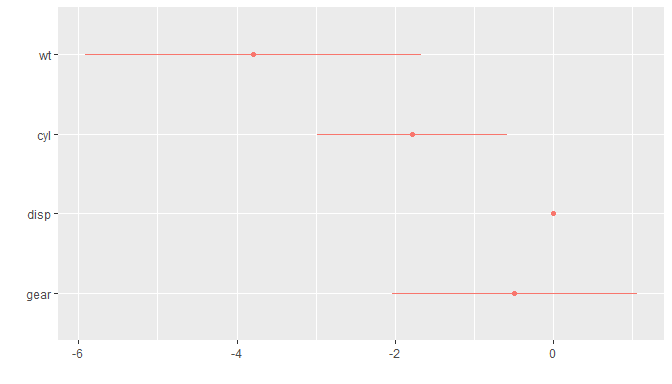If the lower and upper bounds of the uncertainty intervals are already estimated in the model outputs (e.g., in Bayesian analyses), one can ask dwplot to use them by named the columns as conf.low and conf.high. In the following case, we created the conf.low and conf.high based on the std.error. Then you can see, as long as the conf.low and conf.high are defined, dwplot can still replicate the preceding plot when the std.error is removed.

m1a_df <- mutate(m1_df,
conf.low = estimate - qnorm(.975) * std.error,
conf.high = estimate + qnorm(.975) * std.error) %>%
# create the lower and upper bounds
select(-std.error) # remove the std.error

dwplot(m1a_df)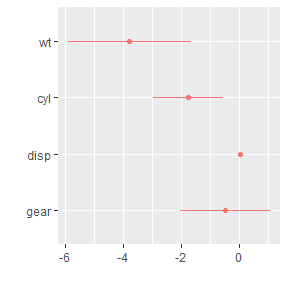Using tidy can be helpful when one wishes to omit certain model estimates from the plot. To illustrate, we drop the intercept:

m1_df <- tidy(m1) %>% filter(term != "gear") %>% mutate(model = "Model 1")
m2_df <- tidy(m2) %>% filter(term != "gear") %>% mutate(model = "Model 2")

two_models <- rbind(m1_df, m2_df)

dwplot(two_models)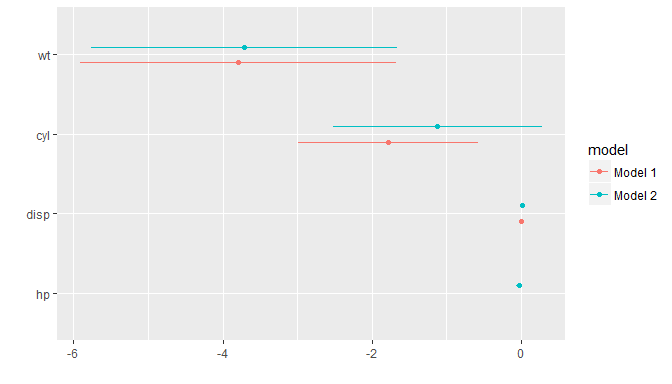It can also be convenient to build a tidy data frame of regression results directly, that is, without first creating model objects:

# Run model on subsets of data, save results as tidy df, make a model variable, and relabel predictors
by_trans <- mtcars %>%
group_by(am) %>%                                         # group data by trans
do(tidy(lm(mpg ~ wt + cyl + disp + gear, data = .))) %>% # run model on each grp
rename(model=am) %>%                                     # make model variable
relabel_predictors(c(wt = "Weight",                      # relabel predictors
cyl = "Cylinder",
disp = "Displacement",
gear = "Gear"))

by_trans
## # A tibble: 10 x 6
## # Groups:   model 
##    model         term     estimate   std.error  statistic      p.value
##    <dbl>        <chr>        <dbl>       <dbl>      <dbl>        <dbl>
##  1     0  (Intercept) 30.742858714  7.41240884  4.1474856 0.0009863882
##  2     0       Weight -2.812158473  1.26617822 -2.2209816 0.0433588317
##  3     0     Cylinder -1.302359700  0.59904454 -2.1740616 0.0473435198
##  4     0 Displacement  0.006919124  0.01294868  0.5343496 0.6014828560
##  5     0         Gear  1.258997239  1.80937061  0.6958205 0.4979303131
##  6     1  (Intercept) 48.377755271 11.14366415  4.3412790 0.0024741252
##  7     1       Weight -7.528674283  2.77396968 -2.7140435 0.0264917835
##  8     1     Cylinder  0.198085116  1.70231059  0.1163625 0.9102333117
##  9     1 Displacement -0.014608455  0.03147434 -0.4641386 0.6549170300
## 10     1         Gear -1.081671599  2.13913305 -0.5056589 0.6267313579
dwplot(by_trans) +
theme_bw() + xlab("Coefficient Estimate") + ylab("") +
geom_vline(xintercept = 0, colour = "grey60", linetype = 2) +
ggtitle("Predicting Gas Mileage by Transmission Type") +
theme(plot.title = element_text(face="bold"),
legend.justification=c(0, 0), legend.position=c(0, 0),
legend.background = element_rect(colour="grey80"),
legend.title.align = .5) +
scale_colour_grey(start = .3, end = .7,
name = "Transmission",
breaks = c(0, 1),
labels = c("Automatic", "Manual"))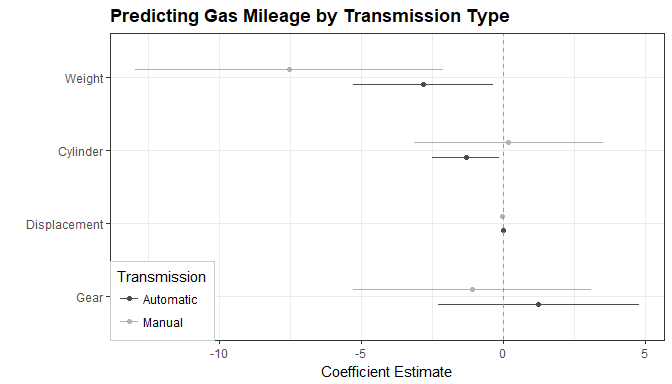Also note in the above example the additional manner of using the relabel_predictors function: in addition to being used on the ggplot object created by dwplot before further customization, it may also be used on a tidy data frame before it is passed to dwplot.

Additionally, one can change the shape of the point estimate instead of using different colors. This can be useful, for example, when a plot needs to be printed in black and white.

 dwplot(by_trans, dot_args = list(aes(colour = model, shape = model), size = .5)) +
theme_bw() + xlab("Coefficient Estimate") + ylab("") +
geom_vline(xintercept = 0, colour = "grey60", linetype = 2) +
ggtitle("Predicting Gas Mileage by Transmission Type") +
theme(plot.title = element_text(face="bold"),
legend.justification=c(0, 0), legend.position=c(0, 0),
legend.background = element_rect(colour="grey80"),
legend.title.align = .5) +
scale_colour_grey(start = .1, end = .1, # if start and end same value, use same colour for all models
name  ="Model",
breaks=c(0, 1),
labels=c("Automatic", "Manual")) +
scale_shape_discrete(name  ="Model",
breaks=c(0, 1),
labels=c("Automatic", "Manual"))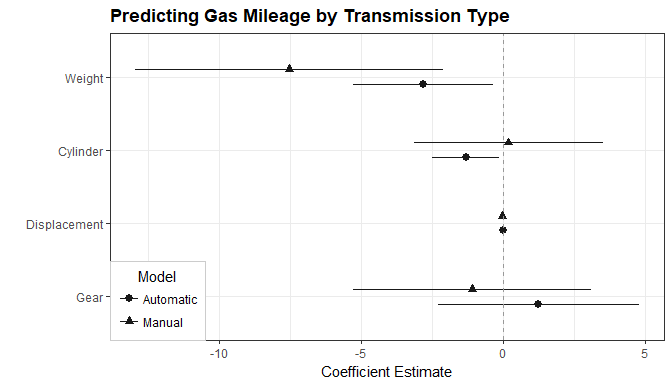It is also easy to plot classes of model objects that are not supported by tidy: one simply extracts the results from the model object and builds the data frame to pass to dwplot oneself. Many functions generate results that can be extracted by coef().

# the ordinal regression model is not supported by tidy
m4 <- ordinal::clm(factor(gear) ~ wt + cyl + disp, data = mtcars)
m4_df <- coef(summary(m4)) %>%
data.frame() %>%
tibble::rownames_to_column("term") %>%
rename(estimate = Estimate, std.error = Std..Error)
m4_df
##   term    estimate  std.error    z.value  Pr...z..
## 1  3|4 -4.03517968 2.45869171 -1.6411898 0.1007580
## 2  4|5 -1.37662018 2.28622404 -0.6021370 0.5470829
## 3   wt -1.13452561 0.98498075 -1.1518252 0.2493929
## 4  cyl  0.41701081 0.60620009  0.6879095 0.4915098
## 5 disp -0.01343896 0.01188167 -1.1310664 0.2580271
dwplot(m4_df)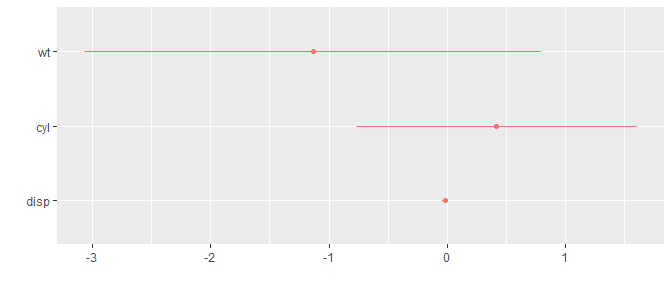Working with a tidy data frame, it is similarly straightforward to plot just a subset of results or to rescale or reorder coefficients. One often desireable manipulation is to standardize the scales of variables. Gelman (2008), for example, suggests rescaling ordinal and continuous predictors by two standard deviations to facilitate comparison with dichotomous predictors. Although this can of course be done before model estimation, it can be more convenient to simply rescale the coefficients afterwards; the by_2sd function, which takes as arguments a data frame of estimates along with the original data frame upon which the model was based, automates this calculation.

# Customize the input data frame
m1_df_mod <- m1_df %>%                 # the original tidy data.frame
by_2sd(mtcars) %>%                 # rescale the coefficients
arrange(term)                      # alphabetize the variables

m1_df_mod  # rescaled, with variables reordered alphabetically
##          term  estimate std.error statistic      p.value   model
## 1 (Intercept) 43.539847  4.860059  8.958708 1.421951e-09 Model 1
## 2         cyl -6.373226  2.192716 -2.906545 7.215756e-03 Model 1
## 3        disp  1.721364  2.976311  0.578355 5.678177e-01 Model 1
## 4          wt -7.422318  2.117028 -3.506008 1.608443e-03 Model 1
dwplot(m1_df_mod)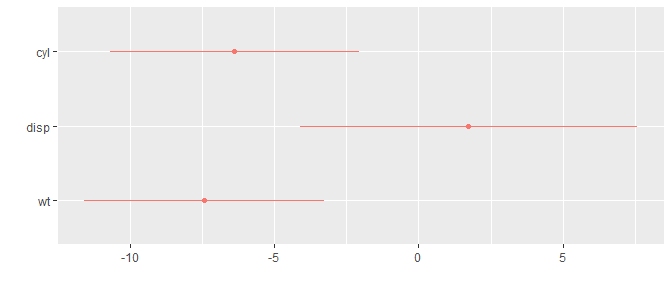An input data frame can also be constructed from estimates of other quantities of interest, such as margins, odds ratios, or predicted probabilities, rather than coefficients.

# Create a data.frame of marginal effects
m5 <- mfx::logitmfx(formula = am ~ wt + cyl + disp, data = mtcars)
m5_margin <- data.frame(m5\$mfxest) %>%
tibble::rownames_to_column("term") %>%
rename(estimate = dF.dx, std.error = Std..Err.)
m5_margin
##   term     estimate   std.error         z      P..z.
## 1   wt -1.121208436 0.591169104 -1.896595 0.05788140
## 2  cyl  0.367534149 0.209932491  1.750725 0.07999322
## 3 disp -0.003335217 0.003148954 -1.059151 0.28953111
dwplot(m5_margin)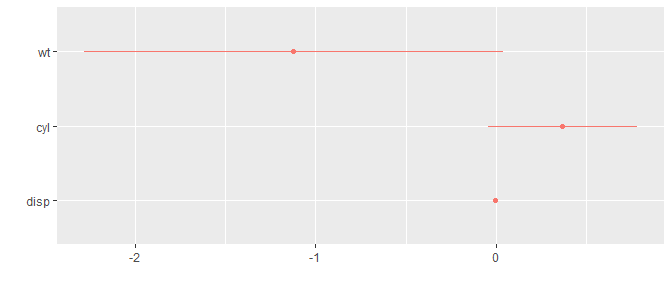## Advanced Use: Grouping Predictors

It is frequently desirable to convey that the predictors in a model depicted in a dot-and-whisker plot form groups of some sort. This can be achieved by passing the finalized plot to the add_brackets function. Note that add_brackets outputs a gtable rather than a ggplot object. Therefore, the output is displayed using grid.arrange and saved using ggsave.

# Define order for predictors that can be grouped
reordered_vars <- c("wt", "cyl", "disp", "hp", "gear", "am")

# Generate a tidy data frame
m123_df <- rbind(tidy(m1) %>% mutate(model = "Model 1"),      # tidy results &
tidy(m2) %>% mutate(model = "Model 2"),      # add a variable to
tidy(m3) %>% mutate(model = "Model 3")) %>%  # identify model.
filter(term != "(Intercept)") %>%                         # remove intercepts
by_2sd(mtcars) %>%                                        # rescale coefficients
mutate(term = factor(term, levels = reordered_vars)) %>%  # make term a factor &
group_by(model) %>% arrange(term) %>%                     # reorder
relabel_predictors(c(wt = "Weight",                       # relabel predictors
cyl = "Cylinders",
disp = "Displacement",
hp = "Horsepower",
gear = "Gears",
am = "Manual"))

# Save finalized plot to an object
p123 <- dwplot(m123_df) +
theme_bw() + xlab("Coefficient Estimate") + ylab("") +
geom_vline(xintercept = 0, colour = "grey60", linetype = 2) +
ggtitle("Predicting Gas Mileage") +
theme(plot.title = element_text(face="bold"),
legend.justification=c(1, 1), legend.position=c(1, 1),
legend.background = element_rect(colour="grey80"),
legend.title = element_blank())

# Create list of brackets (label, topmost included predictor, bottommost included predictor)
three_brackets <- list(c("Overall", "Weight", "Weight"),
c("Engine", "Cylinders", "Horsepower"),
c("Transmission", "Gears", "Manual"))

g123 <- p123 %>% add_brackets(three_brackets)

# to save to file (not run)
# ggsave(file = "plot.pdf", g123)

grid.arrange(g123)    # to display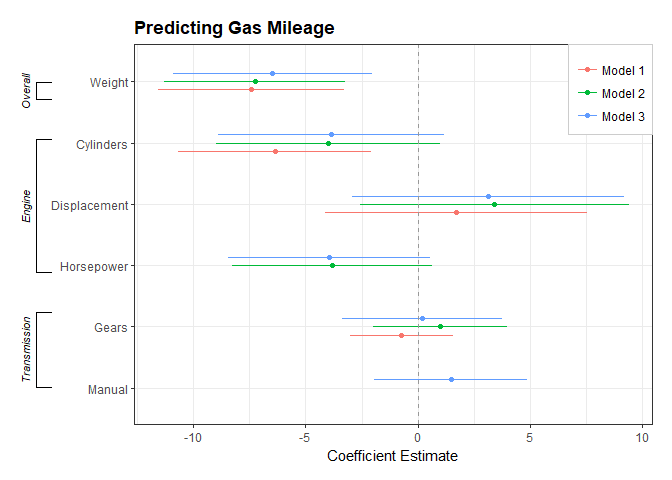## Advanced Use: The ‘Secret Weapon’ and ‘Small Multiple’ Plots

A variation of dot-and-whisker plot is used to compare the estimated coefficients for a single predictor across many models or datasets: Andrew Gelman calls such plots the ‘secret weapon’. They are easy to make with the secret_weapon function. Like dwplot, the function accepts both lists of model objects and tidy data frames as input, and the size of the confidence intervals can be adjusted with the alpha argument. The var argument is used to specify the predictor for which results are to be plotted.

data(diamonds)

# Estimate models for many subsets of data, put results in a tidy data.frame
by_clarity <- diamonds %>% group_by(clarity) %>%
do(tidy(lm(price ~ carat + cut + color, data = .))) %>%
ungroup %>% rename(model = clarity)

# Deploy the secret weapon
secret_weapon(by_clarity, var = "carat", alpha = .01) +
xlab("Estimated Coefficient (Dollars)") + ylab("Diamond Clarity") +
ggtitle("Estimated Coefficients for Diamond Size Across Clarity Grades") +
theme(plot.title = element_text(face="bold"))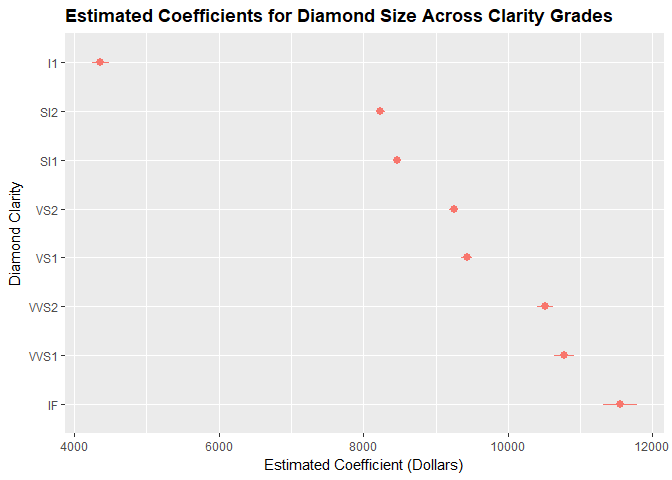A final means of presenting many models’ results at once in a particularly compact format is the “small multiple” plot of regression results (see Kastellec and Leoni 2007, 766). Small-multiple plots present estimates in multiple panels, one for each variable: they are similar to a stack of secret weapon plots. The small_multiple function makes generating these plots simple. Here, we pass a tidy data frame of six models to the function so we can to rescale the coefficients first, but the function can accept a list of model objects as well.

# Generate a tidy data.frame of regression results from six models
m <- list()
ordered_vars <- c("wt", "cyl", "disp", "hp", "gear", "am")
m[] <- lm(mpg ~ wt, data = mtcars)
m123456_df <- m[] %>% tidy %>% by_2sd(mtcars) %>%
mutate(model = "Model 1")
for (i in 2:6) {
m[[i]] <- update(m[[i-1]], paste(". ~ . +", ordered_vars[i]))
m123456_df <- rbind(m123456_df, m[[i]] %>% tidy %>% by_2sd(mtcars) %>%
mutate(model = paste("Model", i)))
}

# Relabel predictors (they will appear as facet labels)
m123456_df <- m123456_df %>%
relabel_predictors(c("(Intercept)" = "Intercept",
wt = "Weight",
cyl = "Cylinders",
disp = "Displacement",
hp = "Horsepower",
gear = "Gears",
am = "Manual"))

# Generate a 'small multiple' plot
small_multiple(m123456_df) +
theme_bw() + ylab("Coefficient Estimate") +
geom_hline(yintercept = 0, colour = "grey60", linetype = 2) +
ggtitle("Predicting Gas Mileage") +
theme(plot.title = element_text(face = "bold"), legend.position = "none",
axis.text.x  = element_text(angle = 60, hjust = 1))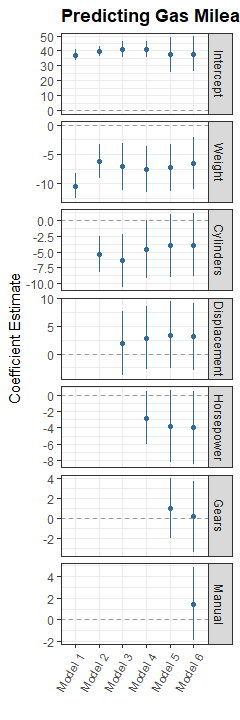To facilitate comparisons across, e.g., results generated across different samples, one can cluster the results presented in a small multiple plot. To do so, results that should be clustered should have the same value of model, but should be assigned different values of an additional submodel variable included in the tidy data frame passed to small_multiple. (We also replicate three examples in Kastellec and Leoni (2007) with dotwhisker in a separate package document, “kl2007_examples”.)

# Generate a tidy data.frame of regression results from five models on
# the mtcars data subset by transmission type
ordered_vars <- c("wt", "cyl", "disp", "hp", "gear")
mod <- "mpg ~ wt"

by_trans <- mtcars %>% group_by(am) %>%  # group data by transmission
do(tidy(lm(mod, data = .))) %>%        # run model on each group
rename(submodel = am) %>%              # make submodel variable
mutate(model = "Model 1") %>%          # make model variable
ungroup()

for (i in 2:5) {
mod <- paste(mod, "+", ordered_vars[i])
by_trans <- rbind(by_trans, mtcars %>% group_by(am) %>%
do(tidy(lm(mod, data = .))) %>%
rename(submodel = am) %>%
mutate(model = paste("Model", i)) %>%
ungroup())
}

# Relabel predictors (they will appear as facet labels)
by_trans <- by_trans %>% dplyr::select(-submodel, everything(), submodel) %>%
relabel_predictors(c("(Intercept)" = "Intercept",
wt = "Weight",
cyl = "Cylinders",
disp = "Displacement",
hp = "Horsepower",
gear = "Gears"))

by_trans
## # A tibble: 40 x 7
##         term   estimate std.error  statistic      p.value   model submodel
##        <chr>      <dbl>     <dbl>      <dbl>        <dbl>   <chr>    <dbl>
##  1 Intercept 31.4160554 2.9467213 10.6613596 6.007748e-09 Model 1        0
##  2    Weight -3.7859075 0.7665567 -4.9388485 1.245595e-04 Model 1        0
##  3 Intercept 46.2944779 3.1198212 14.8388241 1.276849e-08 Model 1        1
##  4    Weight -9.0842680 1.2565727 -7.2294008 1.687904e-05 Model 1        1
##  5 Intercept 34.5680688 2.4820606 13.9271656 2.312644e-10 Model 2        0
##  6    Weight -2.2280342 0.7523460 -2.9614487 9.188908e-03 Model 2        0
##  7 Cylinders -1.2988334 0.3786433 -3.4302297 3.433942e-03 Model 2        0
##  8 Intercept 46.2457474 3.1660012 14.6069896 4.511247e-08 Model 2        1
##  9    Weight -7.4026406 2.3985311 -3.0863226 1.151591e-02 Model 2        1
## 10 Cylinders -0.7889962 0.9532042 -0.8277305 4.271348e-01 Model 2        1
## # ... with 30 more rows
small_multiple(by_trans) +
theme_bw() + ylab("Coefficient Estimate") +
geom_hline(yintercept = 0, colour = "grey60", linetype = 2) +
theme(axis.text.x  = element_text(angle = 45, hjust = 1),
legend.position=c(0.06, 0.02), legend.justification=c(0, 0),
legend.title = element_text(size=8),
legend.background = element_rect(color="gray90"),
legend.margin = unit(-4, "pt"),
legend.key.size = unit(10, "pt")) +
scale_colour_hue(name = "Transmission",
breaks = c(0, 1),
labels = c("Automatic", "Manual")) +
ggtitle("Predicting Gas Mileage\nby Transmission Type")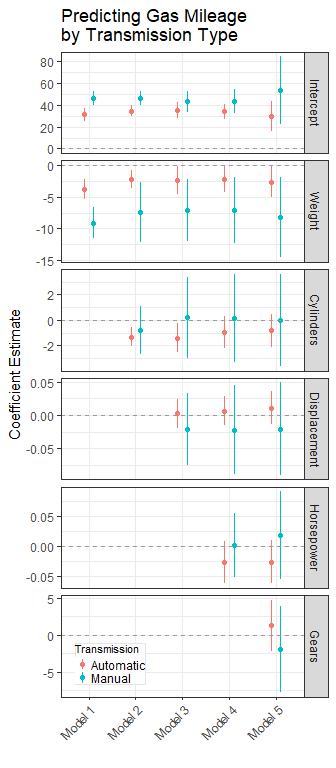## Conclusion

The dotwhisker package provides a flexible and convenient way to visualize regression results and to compare them across models. This vignette offers an overview of its use and features. We encourage users to consult the help files for more details.

## Affiliation

Frederick Solt

Department of Political Science,

University of Iowa,

324 Schaeffer Hall,

20 E Washington St, Iowa City, IA, 52242

Email: frederick-solt@uiowa.edu

Website: http://myweb.uiowa.edu/fsolt

Yue Hu

Department of Political Science,

University of Iowa,

313 Schaeffer Hall,

20 E Washington St, Iowa City, IA, 52242

Email: yue-hu-1@uiowa.edu